# One's and Two's Complement Electrical Engineering (EE) Notes | EduRev

## Electrical Engineering (EE) : One's and Two's Complement Electrical Engineering (EE) Notes | EduRev

The document One's and Two's Complement Electrical Engineering (EE) Notes | EduRev is a part of the Electrical Engineering (EE) Course Digital Electronics.
All you need of Electrical Engineering (EE) at this link: Electrical Engineering (EE)

## Ones Complement

The complement (or opposite) of +5 is −5. When representing positive and negative numbers in 8-bit ones complement binary form, the positive numbers are the same as in signed binary notation described in Number system module i.e. the numbers 0 to +127 are represented as 000000002 to 011111112. However, the complement of these numbers, that is their negative counterparts from −128 to −1, are represented by ‘complementing’ each 1 bit of the positive binary number to 0 and each 0 to 1.

### For example:

+510 is 000001012

−510 is 111110102

Notice in the above example, that the most significant bit (msb) in the negative number −510 is 1, just as in signed binary. The remaining 7 bits of the negative number however are not the same as in signed binary notation. They are just the complement of the remaining 7 bits, and these give the value or magnitude of the number.

The problem with signed the binary arithmetic described in Number system module 1.4  was that it gave the wrong answer when adding positive and negative numbers. Does ones complement notation give better results with negative numbers than signed binary?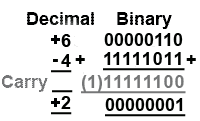##### Fig. 1.5.1 Adding Positive & Negative Numbers in Ones Complement

Fig. 1.5.1 shows the result of adding −4 to +6, using ones complement,(this is the same as subtracting +4 from +6, and so it is crucial to arithmetic).

The result, 000000012 is 110 instead of 210.

This is better than subtraction in signed binary, but it is still not correct. The result should be +210 but the result is +1 (notice that there has also been a carry into the none existent 9th bit).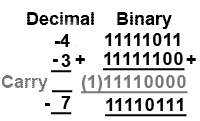Fig. 1.5.2 Adding Positive & Negative Numbers in Ones Complement

Fig. 1.5.2 shows another example, this time adding two negative numbers −4 and −3. Because both numbers are negative, they are first converted to ones complement notation. +410 is 00000100 in pure 8 bit binary so complementing gives 11111011.

This is −410 in ones complement notation.

+310 is 00000011 in pure 8 bit binary, so complementing gives 11111100.

This is −310 in ones complement notation.

The result of 111101112 is in its complemented form so the 7 bits after the sign bit (1110111), should be re-complemented and read as 0001000, which gives the value 810. As the most significant bit (msb) of the result is 1 the result must be negative, which is correct, but the remaining seven bits give the value of −8. This is still wrong by 1, it should be −7.

### End Around Carry

There is a way to correct this however. Whenever the ones complement system handles negative numbers, the result is 1 less than it should be, e.g. 1 instead of 2 and −8 instead of −7, but another thing that happens in negative number ones complement calculations is that a carry is ‘left over’ after the most significant bits are added. Instead of just disregarding this carry bit, it can be added to the least significant bit of the result to correct the value. This process is called ‘end around carry’ and corrects for the result -1 effect of the ones complement system.

There are however, still problems with both ones complement and signed binary notation. The ones complement system still has two ways of writing 010 (000000002 = +010 and 111111112= −010); additionally there is a problem with the way positive and negative numbers are written. In any number system, the positive and negative versions of the same number should add to produce zero. As can be seen from Table 1.5.1, adding +45 and −45 in decimal produces a result of zero, but this is not the case in either signed binary or ones complement.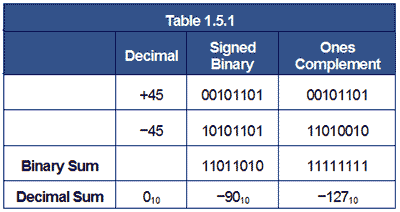This is not good enough, however there is a system that overcomes this difficulty and allows correct operation using both positive and negative numbers. This is the Twos Complement system.

## Twos Complement Notation

Twos complement notation solves the problem of the relationship between positive and negative numbers, and achieves accurate results in subtractions.

To perform binary subtraction, the twos complement system uses the technique of complementing the number to be subtracted. In the ones complement system this produced a result that was 1 less than the correct answer, but this could be corrected by using the ‘end around carry’ system. This still left the problem that positive and negative versions of the same number did not produce zero when added together.

The twos complement system overcomes both of these problems by simply adding one to the ones complement version of the number before addition takes place. The process of producing a negative number in Twos Complement Notation is illustrated in Table 1.5.2.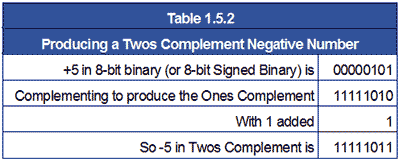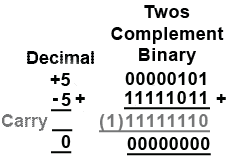Fig. 1.5.3 Adding a Number to its Twos Complement Produces Zero

This version of −5 now, not only gives the correct answer when used in subtractions but is also the additive inverse of +5 i.e. when added to +5 produces the correct result of 0, as shown in Fig. 1.5.3

Note that in twos complement the (1) carry from the most significant bit is discarded as there is no need for the ‘end around carry’ fix.

With numbers electronically stored in their twos complement form, subtractions can be carried out more easily (and faster) as the microprocessor has simply to add two numbers together using nearly the same circuitry as is used for addition.

6 − 2 = 4 is the same as (+6) + (−2) = 4

## Twos Complement Examples

Note: When working with twos complement it is important to write numbers in their full 8 bit form, since complementing will change any leading 0 bits into 1 bits, which will be included in any calculation. Also during addition, carry bits can extend into leading 0 bits or sign bits, and this can affect the answer in unexpected ways.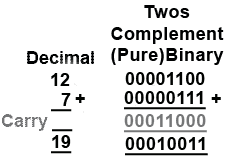Fig. 1.5.4 Adding Positive Numbers in Twos Complement

Fig 1.5.4 shows an example of addition using 8 bit twos complement notation. When adding two positive numbers, their the sign bits (msb) will both be 0, so the numbers are written and added as a pure 8-bit binary addition.

### Twos Complement Subtraction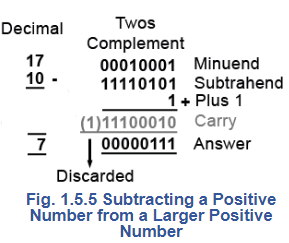Fig. 1.5.5 shows the simplest case of twos complement subtraction where one positive number (the subtrahend) is subtracted from a larger positive number (the minuend). In this case the minuend is 1710 and the subtrahend is 1010.

Because the minuend is a positive number its sign bit (msb) is 0 and so it can be written as a pure 8 bit binary number.

The subtrahend is to be subtracted from the minuend and so needs to be complemented (simple ones complement) and 1 added to the least significant bit (lsb) to complete the twos complement and turn +10 into −10.

When these three lines of digits, and any carry 1 bits are added, remembering that in twos complement, any carry from the most significant bit is discarded. The answer (the difference between 17 and 10) is 000001112 = 710 which is correct. Therefore the twos complement method has provided correct subtraction by using only addition and complementing, both operations that can be simply accomplished by digital electronic circuits.

### Subtraction with a negative result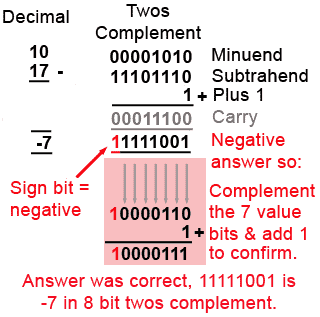##### Fig. 1.5.6 Subtraction Producing a Negative Result

Some subtractions will of course produce an answer with a negative value. In Fig. 1.5.6 the result of subtracting 17 from 10 should −710 but the twos complement answer of 111110012 certainly doesn’t look like −7. However the sign bit is indicating correctly that the answer is negative, so in this case the 7 bits indicating the value of the negative answer need to be 'twos complemented' once more to see the answer in a recognisable form.

When the 7 value bits are complemented and 1 is added to the least significant bit however, like magic, the answer of 100001112 appears, which confirms that the original answer was in fact −7 in 8 bit twos complement form.

It seems then, that twos complement will get the right answer in every situation?

Well guess what − it doesn’t! There are some cases where even twos complement will give a wrong answer. In fact there are four conditions where a wrong answer may crop up:

1. When adding large positive numbers.

2. When adding large negative numbers.

3. When subtracting a large negative number from a large positive number.

4. When subtracting a large positive number from a large negative number.

The problem seems to be with the word ‘large’. What is large depends on the size of the digital word the microprocessor uses for calculation. As shown in Table 1.5.3, if the microprocessor uses an 8−bit word, the largest positive number that can appear in the problem OR THE RESULT is +12710 and the largest negative number will be −12810. The range of positive values appears to be 1 less than the negative range because 0 is a positive number in twos complement and has only one occurrence (000000002) in the whole range of 25610 values.

With a 16-bit word length the largest positive and negative numbers will be +3276710 and -3276810, but there is still a limit to the largest number that can appear in a single calculation.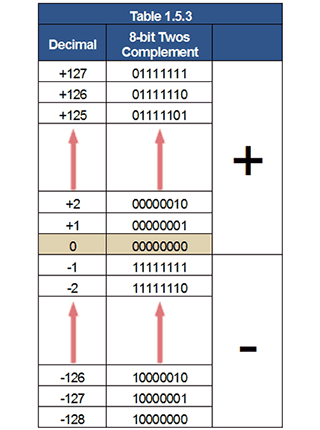### Overflow Problems.

Steps can be taken to accommodate large numbers, by breaking a long binary word down into byte sized sections and carrying out several separate calculations before assembling the final answer. However this doesn’t solve all the cases where errors can occur.

A typical overflow problem that can happen even with single byte numbers is illustrated in Fig. 1.5.7.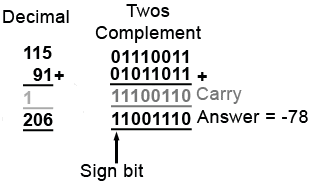##### Fig. 1.5.7 Carry Overflows into Sign Bit

In this example, the two numbers to be added (11510 and 9110) should give a sum of 20610 and at first glance 110011102 looks like the correct answer of 20610,but remember that in the 8 bit twos complement system the most significant bit is the sign of the number, therefore the answer appears to be a negative value and reading just the lower 7 bits gives 10011102 or -7810. Although twos complement negative answers are not easy to read, this is clearly wrong as the result of adding two positive numbers must give a positive answer.

According to the information in Fig 1.5.6, as the answer is negative, complementing the lower 7 bits of 110011102 and adding 1 should reveal the value of the correct answer, but carrying out the complement+1 on these bits and leaving the msb unchanged gives 101100102 which is -5010. This is nothing like the correct answer of 20610 so what has happened?

The 8 bit twos complement notation has not worked here because adding 115 + 91 gives a total greater than +127, the largest value that can be held in 8-bit twos complement notation.

What has happened is that an overflow has occurred, due to a 1 being carried from bit 6 to bit 7 (the most significant bit, which is of course the sign bit), this changes the sign of the answer. Additionally it changes the value of the answer by 12810 because that would be the value of the msb in pure binary. So the original answer of 7810 has ‘lost’ 12810 to the sign bit. The addition would have been correct if the sign bit had been part of the value, however the calculation was done in twos complement notation and the sign bit is not part of the value.

Of course in real electronic calculations, a single byte overflow situation does not usually cause a problem; computers and calculators can fortunately deal with larger numbers than 12710. They achieve this because the microprocessors used are programmed to carry out the calculation in a number of steps, and although each step must still be carried out in a register having a set word length, e.g. 8 bits or 16 bits, corrective action can also be taken if an overflow situation is detected at any stage.

Microprocessors deal with this problem by using a special register called a status register, flag register or conditions code register, which automatically flags up any problem such as an overflow or a change of sign, that occurs. It also provides other information useful to the programmer, so that whatever problem occurs; corrective action can be taken by software, or in many cases by firmware permanently embedded within the microprocessor to deal with a range of math problems.

Whatever word length the microprocessor is designed to handle however, there must always be a limit to the word length, and so the programmer must be aware of the danger of errors similar to that described in Fig. 1.5.7.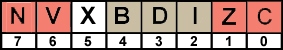##### Fig. 1.5.8 Typical 8-bit Flag Register

A typical flag register is illustrated in Fig. 1.5.8 and consists of a single 8-bit storage register located within the microprocessor, in which some bits may be set by software to control the actions of the microprocessor, and some bits are set automatically by the results of arithmetic operations within the microprocessor.

### Typical flags for an 8-bit microprocessor are listed below:

Bit 0 (C) (set by arithmetic result) = 1 Carry has been created from result msb.

Bit 1 (Z) (set by arithmetic result) = 1 Calculation resulted in 0.

Bit 2 (I) (set by software) 1 = Interrupt disable (Prevents software interrupts).

Bit 3 (D) (set by software) 1 = Decimal mode (Calculations are in BCD).

Bit 4 (B) (set by software) 1 = Break (Stops software execution).

Bit 5 (X) Not used.

Bit 6 (V) (set by arithmetic result) = 1 Overflow has occurred (result too big for 8 bits).

Bit 7 (N) (set by arithmetic result) = 1 Negative result (msb of result is 1).

It seems therefore, that the only math that microprocessors can do is to add together two numbers of a limited value, and to complement binary numbers. Well at a basic level this is true, however there are some additional tricks they can perform, such as shifting all the bits in a binary word left or right, as a partial aid to multiplication or division. However anything more complex must be done by software.

## Multiplication and Division

While addition and subtraction can be achieved by adding positive and negative numbers as described above, this does not include the other basic forms of mathematics, multiplication and division. Multiplication in its simplest form can however be achieved by adding a number to itself a number of times, for example, starting with a total of 0, if 5 is added to the total three times the new total will be fifteen (or 5 x 3). Division can also be accomplished by repeatedly subtracting (using add) the divisor from the number to be divided until the remainder is zero, or less than the divisor. Counting the number of subtractions then gives the result, for example if 3 (the divisor) is repeatedly subtracted from 15, after 5 subtractions the remainder will be zero and the count will be 5, indicating that 15 divided by 3 is exactly 5.

There are more efficient methods for carrying out subtraction and division using software, or extra features within some microprocessors and/or the use of embedded maths firmware.

Offer running on EduRev: Apply code STAYHOME200 to get INR 200 off on our premium plan EduRev Infinity!

## Digital Electronics

91 videos|75 docs|8 tests

,

,

,

,

,

,

,

,

,

,

,

,

,

,

,

,

,

,

,

,

,

;